11.0. Test Results: Part B

11.1.1. Test 1: Test RS232 Communications (TX Only)
11.1.2. Test 2: Fully Test RS232 Communications
11.1.4. Conclusion
11.2. Test Session 2: FG Wilson Lab (05/03/2002)
11.2.1. Test 4: Test RS232 Communications (Baud Rate Set by DIP Switches)
11.2.2. Test 5: Fully Test RS232 Communications (Baud Rate Set by DIP Switches)
11.2.3. Test 6: Test ADC, Scope Program and Real-time Communication Protocol
11.2.4. Test 7: Test Timer Interrupts
11.2.5. Conclusion

11.3. Test Session 3: FG Wilson Lab (22/03/2002)

11.3.1. Test 8: Dual Channel Test

Program mark8.c was compiled and loaded into the PIC. Basically this program chops between channel 1 and channel 2 (e.g. sample CH1, trans, sample CH2, trans, delay, repeat). The test was a complete success the scope.exe program was able to display both channels correctly. Allow big triggering problem, e.g. if channel 1 was selected for trigger it was almost impossible to get a stable channel 2 trace, and if channel 2 was selected for trigger it was difficult to get a stable channel 1 trace. This is a software problem and should be fixable.

Screen dump of the scope program (version 1.036, 19/03/2002) during this dual channel test, is shown in figure 11.3.1a.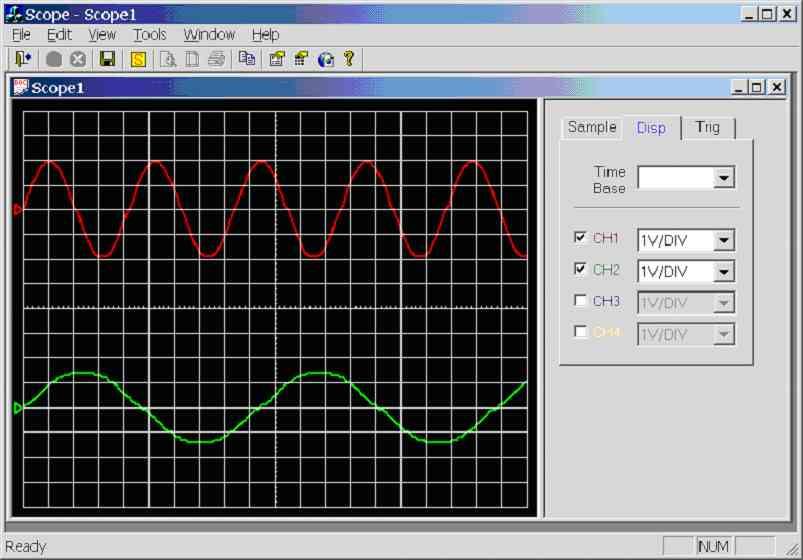Figure 11.3.1a. Screen dump of scope program (Dual Channel Test)

11.3.2. Test 9: Interrupt Driven Time-Base, Real-Time Sampling (Four Channels)

Program mark9.c was compiled and loaded into the PIC. This program uses timer 2 (PIC built-in timer) as the time-base, the timer causes an interrupt every 20µS. The variable ‘TimeBaseMUX’ is the time – base multiplier (e.g. 100 Hz = 10ms, hence TimeBaseMUX = 500). Note no sampling is done during the interrupt routine as all interrupts are disabled while an interrupt is being processed, hence the interrupt routine needs to take less than 20µS to execute or timing would inaccurate (e.g. say the interrupt routine execute time was longer than 20µS, during this time timer 2 cannot cause any more interrupts, hence accurate timing is lost). The main program loops continuously checking the ‘bSample’ flag, if the flag is true the fuctuion Sample_RealTime() is called, else it continues to loop. The ‘bSample’ flag is set in the Time-base interrupt routine, keeping the interrupt short.

Interrupt driven time-base has one big advantage over using preset delays: delay routines do not take into consideration processing delays (e.g. waiting for UART buffer to empty), while the PICs real-time timer will continue to count no matter what the PIC is doing, and will cause an interrupt every 20µS (assuming interrupt routine is finished before it is time to called it again).

This program samples all four channels (chop mode). E.g. channel 1 is sampled, then channel 2, then channel 3, then channel 4. It is expected that Alt mode will be added some time in the future, e.g. sample channel 1 a thousand times, then sample channel 2 a thousand times, etc...

The following experiments were carried out with the TimeBaseMux equal to 50, that’s a time-base of 1000Hz (1/(50*20µS)) and baud rate was set at 115kbps. Scope.exe (version 1.036 - 19/03/2002) was used and a calibrated function generator (Fluke PM5139) used to generate input signals.

Note: Sample rate = 1000Hz, 10 horizontal, samples per division. Hence scope time-base is 0.01 seconds per division. Time-base is fixed on this version of the scope program.

25Hz sinewave (0-5V) connected to CH1/AN0 and all other channels connected to 5V, see figure 11.3.2a. 4 divisions per cycle, hence the calculated channel 1 frequency is 1/(4*0.01) = 25Hz. Channels 2, 3 & 4 are at 5V DC.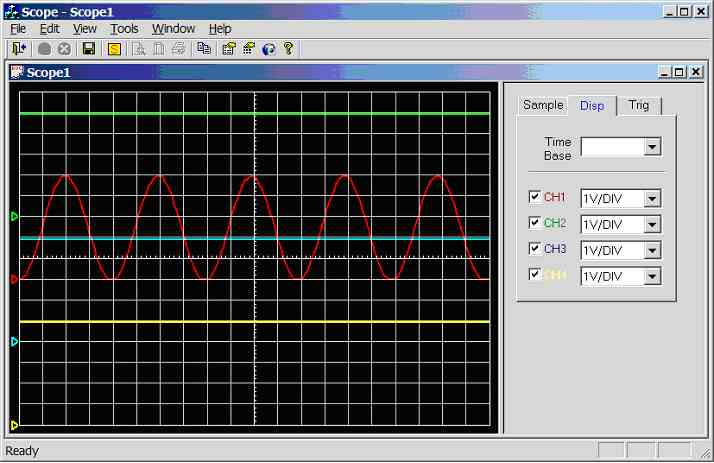Figure 11.3.2a. Screen dump of scope program (sample rate = 1 kHz, CH1 = 25Hz sinewave, CH2 = 5V, CH3 = 5V, CH4 = 5V)

25Hz sinewave (0-5V) connected to CH2/AN1 and all other channels connected to 5V, see figure 11.3.2b. 4 divisions per cycle, hence the calculated channel 2 frequency is 1/(4*0.01) = 25Hz. Channels 1, 3 & 4 are at 5V DC.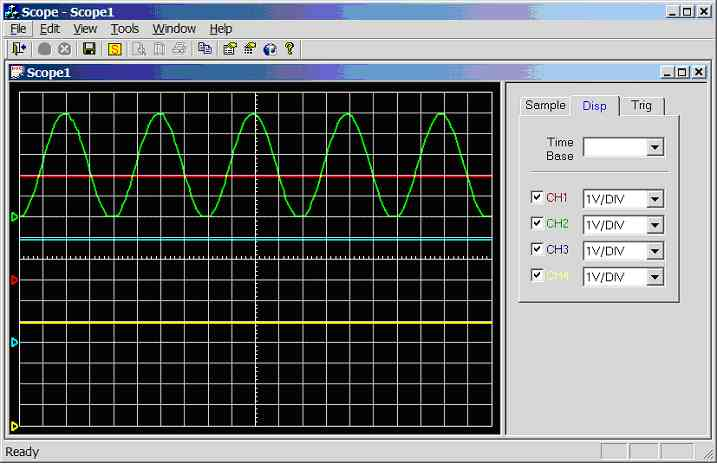Figure 11.3.2b. Screen dump of scope program (sample rate = 1 kHz, CH2 = 25Hz sinewave, CH1 = 5V, CH3 = 5V, CH4 = 5V)

25Hz sinewave (0-5V) connected to CH3/AN2 and all other channels connected to 5V, see figure 11.3.2c. 4 divisions per cycle, hence the calculated channel 3 frequency is 1/(4*0.01) = 25Hz. Channels 1, 2 & 4 are at 5V DC.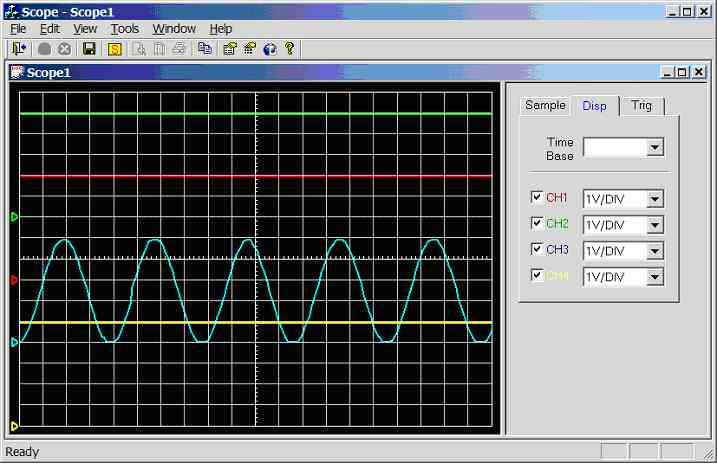Figure 11.3.2c. Screen dump of scope program (sample rate = 1 kHz, CH3 = 25Hz sinewave, CH1 = 5V, CH2 = 5V, CH4 = 5V)

25Hz sinewave (0-5V) connected to CH4/AN3 and all other channels connected to 5V, see figure 11.3.2d. 4 divisions per cycle, hence the calculated channel 4 frequency is 1/(4*0.01) = 25Hz. Channels 1, 2 & 3 are at 5V DC.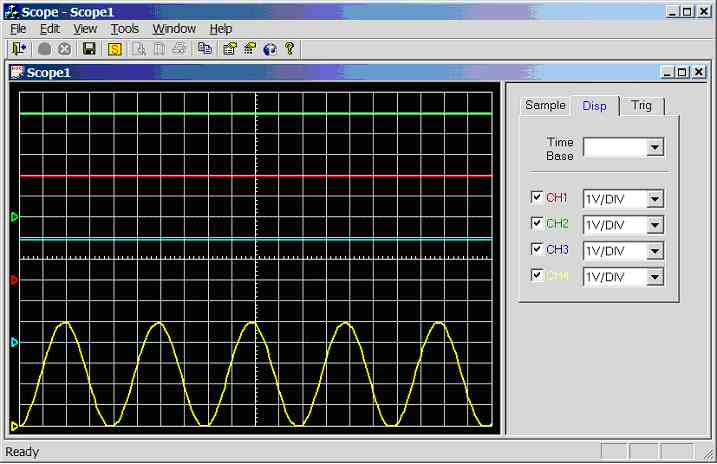Figure 11.3.2d. Screen dump of scope program (sample rate = 1 kHz, CH4 = 25Hz sinewave, CH1 = 5V, CH2 = 5V, CH3 = 5V)

11.3.2.1. Dual Channel Operation Using Two Signal Generators

Fluke PM5139 function generator (calibrated) connected to channel 1 (red), Thandar TG 101 function generator (not calibrated) connected to channel 2 (green).  Sample rate is 1000Hz (scope time-base is 0.01).

Channel 1 connected to 10Hz sine-wave (5Vpp), channel 2 connected to 30Hz sine-wave (4Vpp), see figure 11.3.2.1a. Channel 1: 10 divisions per cycle, hence the calculated frequency is 1/(10*0.01) = 10Hz. Channel 2: 4.2 divisions per cycle, hence the calculated frequency is 1/(4.2*0.01) = 23.8 Hz (note channel 2 signal generator is not calibrated, this is the true frequency).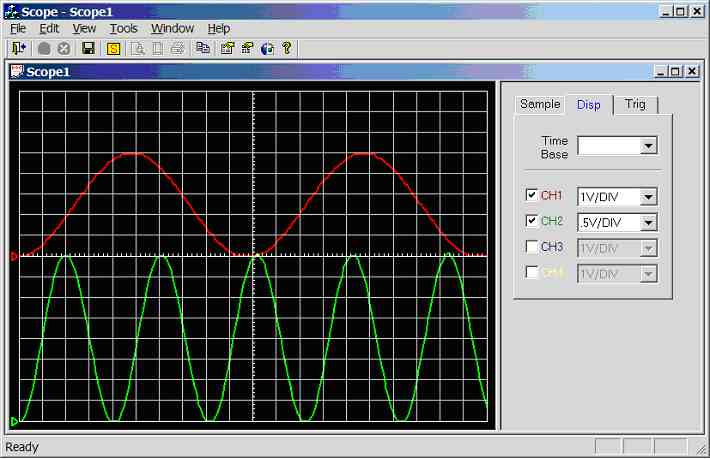Figure 11.3.2.1a. Screen dump of scope program (sample rate = 1 kHz, CH1 = 10Hz sinewave, CH2 = 30Hz sinewave)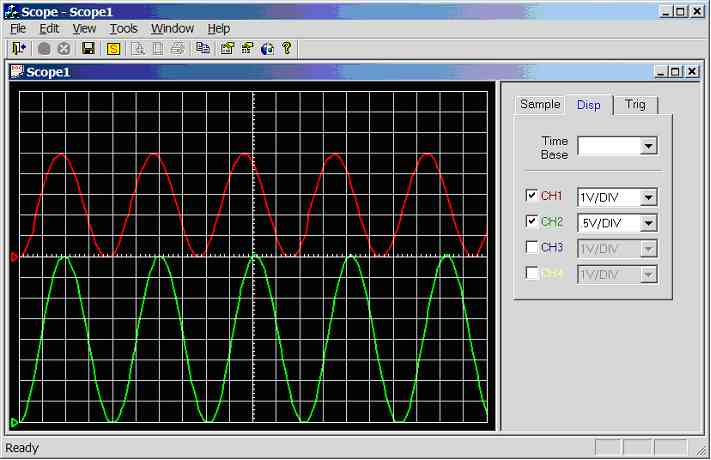Figure 11.3.2.1b. Screen dump of scope program (sample rate = 1 kHz, CH1 = 25Hz sinewave, CH2 = 30Hz sinewave)

Channel 1 connected to 25Hz sine-wave (5Vpp), channel 2 connected to 30Hz sine-wave (4Vpp), see figure 11.3.2.1b. Channel 1: 4 divisions per cycle, hence the calculated frequency is 1/(4*0.01) = 25Hz. Channel 2 unaffected.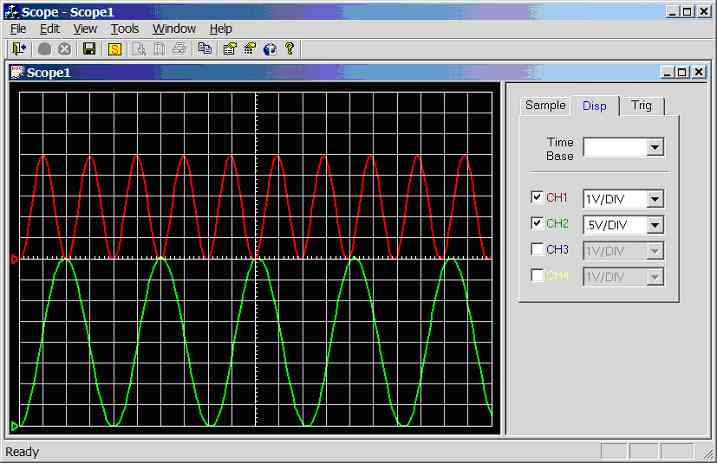Figure 11.3.2.1c. Screen dump of scope program (sample rate = 1 kHz, CH1 = 50Hz sinewave, CH2 = 30Hz sinewave)

Channel 1 connected to 50Hz sine-wave (5Vpp), channel 2 connected to 30Hz sine-wave (4Vpp), see figure 11.3.2.1c. Channel 1: 2 divisions per cycle, hence the calculated frequency is 1/(2*0.01) = 50Hz. Channel 2 unaffected.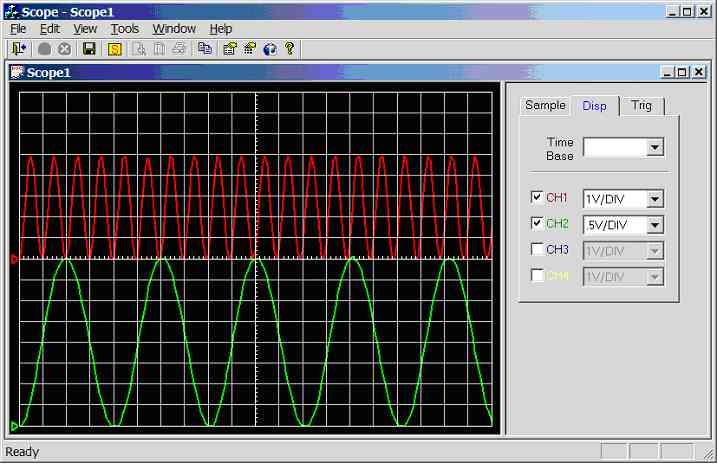Figure 11.3.2.1d. Screen dump of scope program (sample rate = 1 kHz, CH1 = 100Hz sinewave, CH2 = 30Hz sinewave)

Channel 1 connected to 100Hz sine-wave (5Vpp), channel 2 connected to 30Hz sine-wave (4Vpp), see figure 11.3.2.1d. Channel 1: 1 divisions per cycle, hence the calculated frequency is 1/(1*0.01) = 100Hz. Channel 2 unaffected.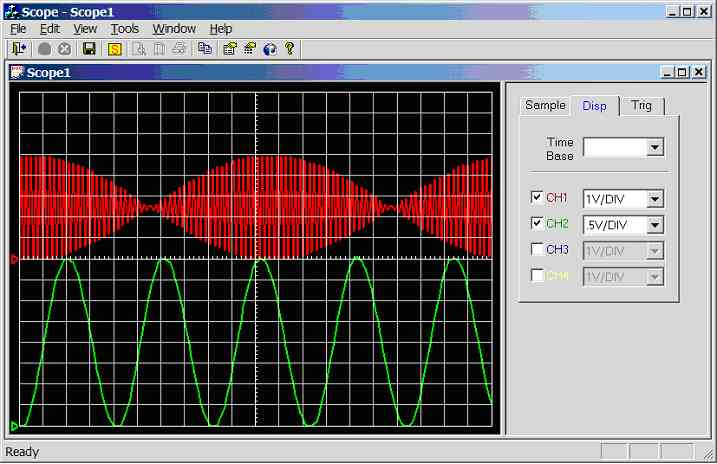Figure 11.3.2.1e. Screen dump of scope program (sample rate = 1 kHz, CH1 = 500Hz sinewave, CH2 = 30Hz sinewave)

Channel 1 connected to 500Hz sine-wave (5Vpp), channel 2 connected to 30Hz sine-wave (4Vpp), see figure 11.3.2.1e. Channel 1: Under sampled, aliasing has occurred. Channel 2 unaffected.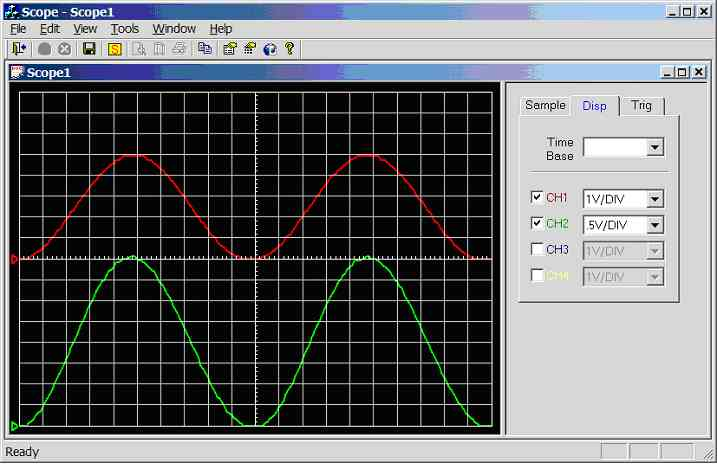Figure 11.3.2.1f. Screen dump of scope program (sample rate = 1 kHz, CH1 = 500Hz sinewave, CH2 = 30Hz sinewave)

Channel 1 connected to 10Hz sine-wave (5Vpp), channel 2 connected to 10Hz sine-wave (4Vpp), see figure 11.3.2.1f. Channel 1: 10 divisions per cycle, hence the calculated frequency is 1/(10*0.01) = 10Hz. Channel 2: 4.2 divisions per cycle, hence the calculated frequency is 1/(10*0.01) = 10 Hz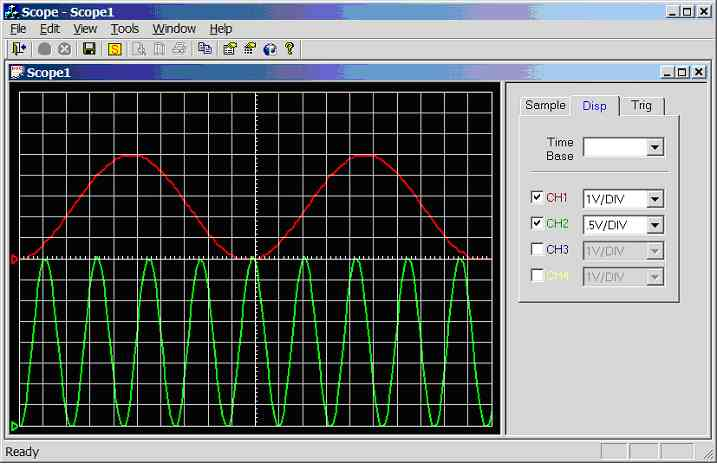Figure 11.3.2.1g. Screen dump of scope program (sample rate = 1 kHz, CH1 = 10Hz sinewave, CH2 = 50Hz sinewave)

Channel 1 connected to 10Hz sine-wave (5Vpp), channel 2 connected to 50Hz sine-wave (4Vpp), see figure 11.3.2.1g. Channel 1: unaffected. Channel 2: 2 divisions per cycle, hence the calculated frequency is 1/(2*0.01) = 50 Hz.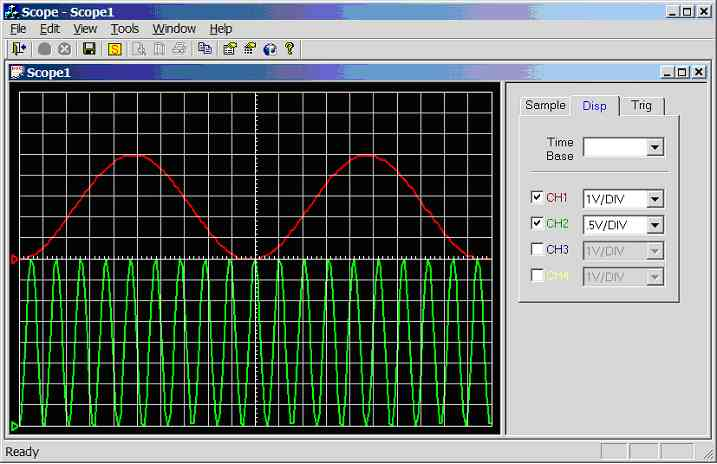Figure 11.3.2.1h. Screen dump of scope program (sample rate = 1 kHz, CH1 = 10Hz sinewave, CH2 = 100Hz sinewave)

Channel 1 connected to 10Hz sine-wave (5Vpp), channel 2 connected to 100Hz sine-wave (4Vpp), see figure 11.3.2.1h. Channel 1: unaffected. Channel 2: 2 divisions per cycle, hence the calculated frequency is 1/(1*0.01) = 100 Hz.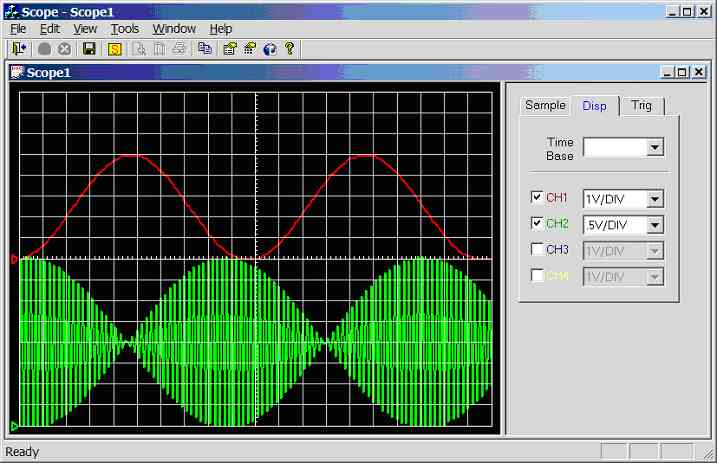Figure 11.3.2.1i. Screen dump of scope program (sample rate = 1 kHz, CH1 = 10Hz sinewave, CH2 = 550Hz sinewave)

Channel 1 connected to 10Hz sine-wave (5Vpp), channel 2 connected to 550Hz sine-wave (4Vpp), see figure 11.3.2.1i. Channel 1: unaffected. Channel 2: Under sampled, aliasing has occurred.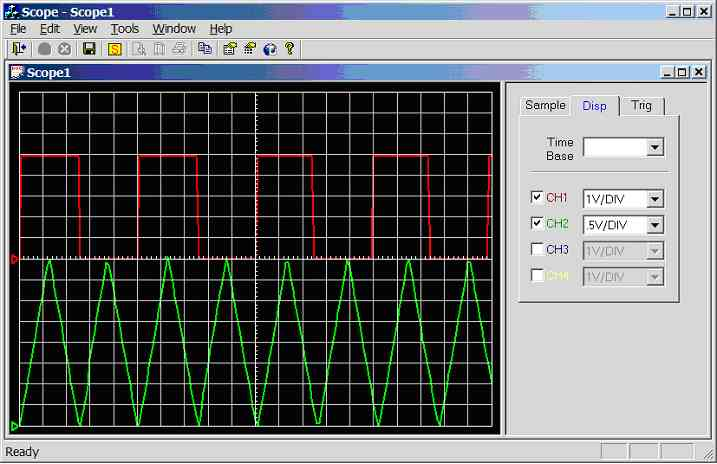Figure 11.3.2.1j. Screen dump of scope program (sample rate = 1 kHz, CH1 = square wave, CH2 = trianglewave)

Note all of the experiments (in this section) were carried using independent triggering mode, because it was difficult to get two stable traces when triggering off one channel (e.g. if triggering on CH1, CH2 is unstable, and if triggering on CH2, CH1 was unstable). This problem should be software fixable.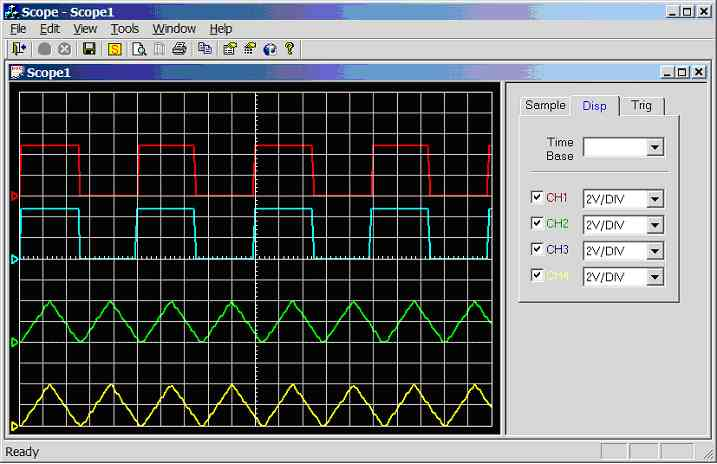Figure 11.3.2.2a. Screen dump of scope program (CH1 = CH2 = squarewave, CH3 = CH4 = trianglewave)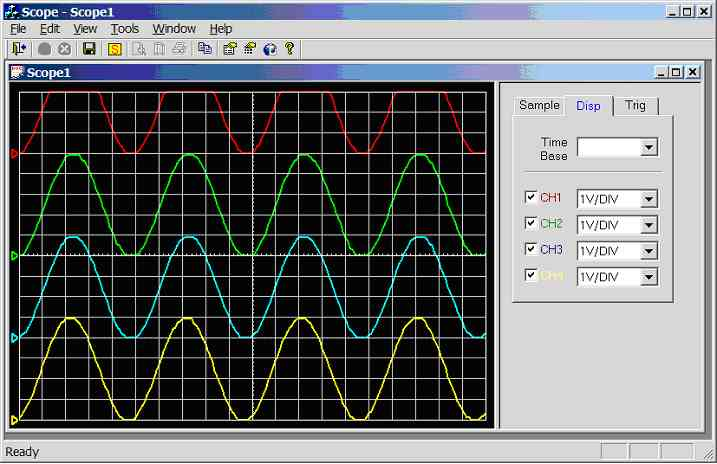Figure 11.3.2.2b. Screen dump of scope program (CH1 = CH2 = CH3 = CH4 = sinewave, triggering on CH1)

All waveforms in figure 11.3.2.2b were stable, note that there should be a 20µS delay (see mark9.c) between each waveform (e.g. chop mode). For example channel 1 should lead channel 2 by 20µS, channel 2 should lead channel 3 by 20µS etc… Each square represents 0.01 seconds (10mS); hence this small offset is not noticeable at this resolution.

11.3.2.3. 5000Hz Sample Rate – Single Channel

mark9.c modified, basically the sample rate has been changed to 5000Hz (TimeBaseMUX = 10), and sampling of channels 2 to 4 has been removed.

Note: Sample rate = 5000Hz, 10 horizontal, samples per division. Hence scope time-base is 2 milliseconds per division. Time-base is fixed on this version of the scope program.

50Hz sinewave (0-5V) connected to CH1 (AN0), see figure 11.3.2.3a. 10 divisions per cycle, hence the calculated frequency is 1/(10*0.002) = 50Hz.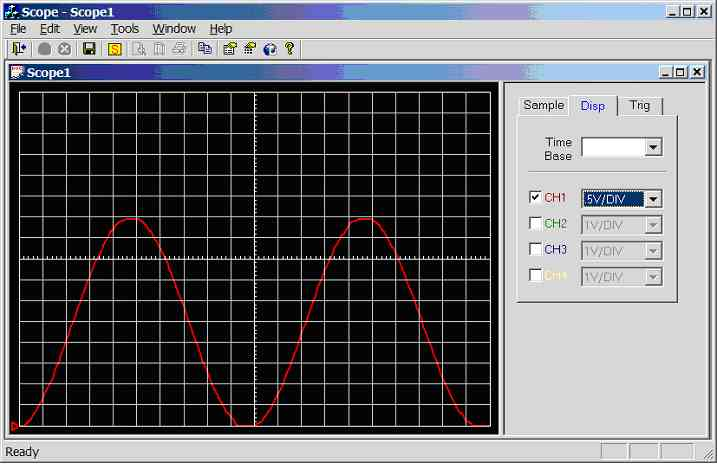Figure 11.3.2.3a. Screen dump of scope program (sample rate = 5000Hz, input wave = 50Hz sinewave 0-5V)

100Hz sinewave (0-5V) connected to CH1 (AN0), see figure 11.3.2.3b. 5 divisions per cycle, hence the calculated frequency is 1/(5*0.002) = 100Hz.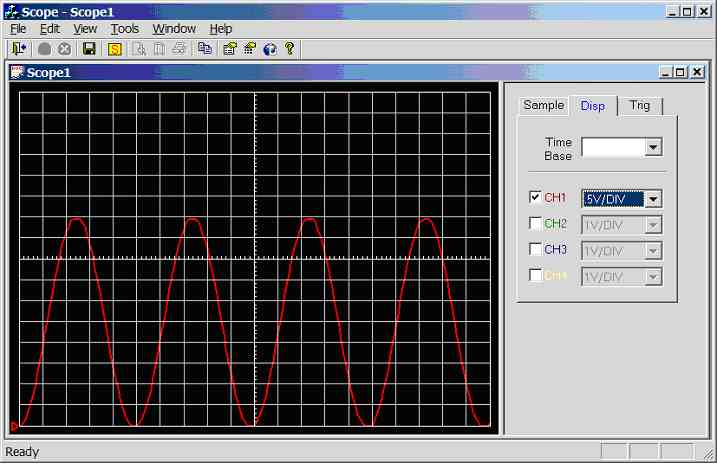Figure 11.3.2.3b. Screen dump of scope program (sample rate = 5000Hz, input wave = 100Hz sinewave 0-5V)

200Hz sinewave (0-5V) connected to CH1 (AN0), see figure 11.3.2.3c. 2.5 divisions per cycle, hence the calculated frequency is 1/(2.5*0.002) = 200Hz.Figure 11.3.2.3c. Screen dump of scope program (sample rate = 5000Hz, input wave = 200Hz sinewave 0-5V)

300Hz sinewave (0-5V) connected to CH1 (AN0), see figure 11.3.2.3d. 1.7 divisions per cycle, hence the calculated frequency is 1/(1.7*0.002) = 294.1Hz.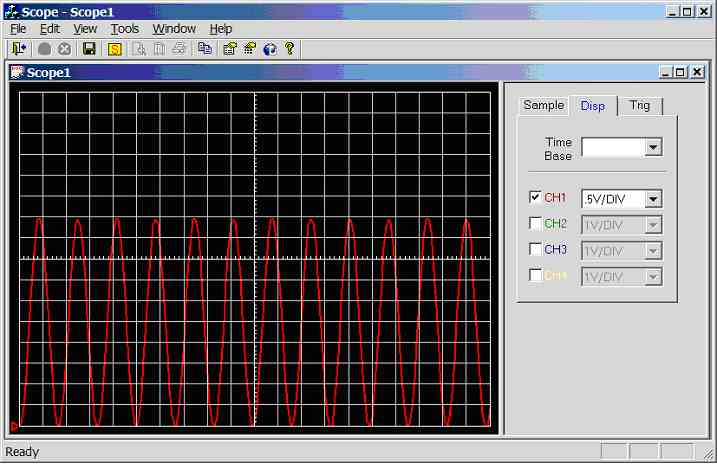Figure 11.3.2.3d. Screen dump of scope program (sample rate = 5000Hz, input wave = 300Hz sinewave 0-5V)

400Hz sinewave (0-5V) connected to CH1 (AN0), see figure 11.3.2.3e. 1.3 divisions per cycle, hence the calculated frequency is 1/(1.3 *0.002) = 384.6Hz.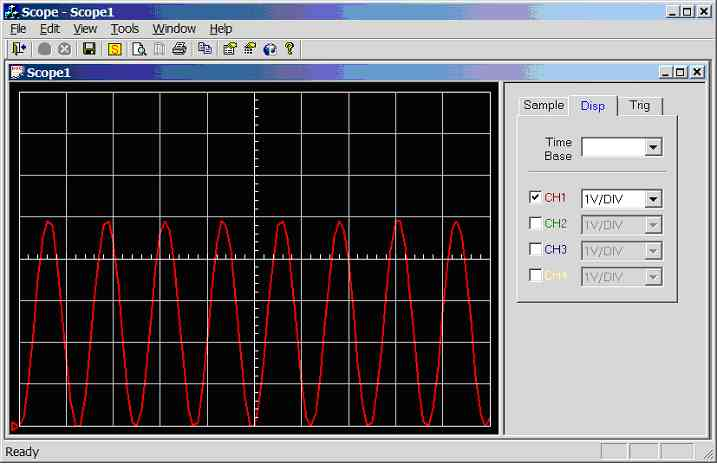Figure 11.3.2.3e. Screen dump of scope program (sample rate = 5000Hz, input wave = 400Hz sinewave 0-5V)

500Hz sinewave (0-5V) connected to CH1 (AN0), see figure 11.3.2.3f. 1 divisions per cycle, hence the calculated frequency is 1/(1 *0.002) = 500Hz.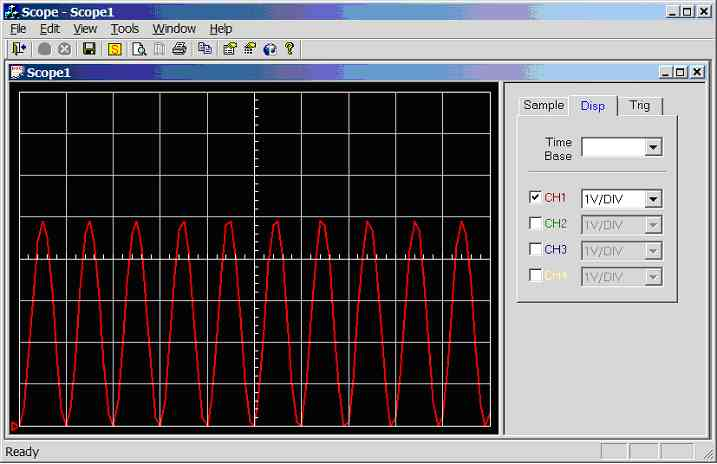Figure 11.3.2.3f. Screen dump of scope program (sample rate = 5000Hz, input wave = 500Hz sinewave 0-5V)

750Hz sinewave (0-5V) connected to CH1 (AN0), see figure 11.3.2.3g. 0.7 divisions per cycle, hence the calculated frequency is 1/(0.7 *0.002) = 714.2Hz.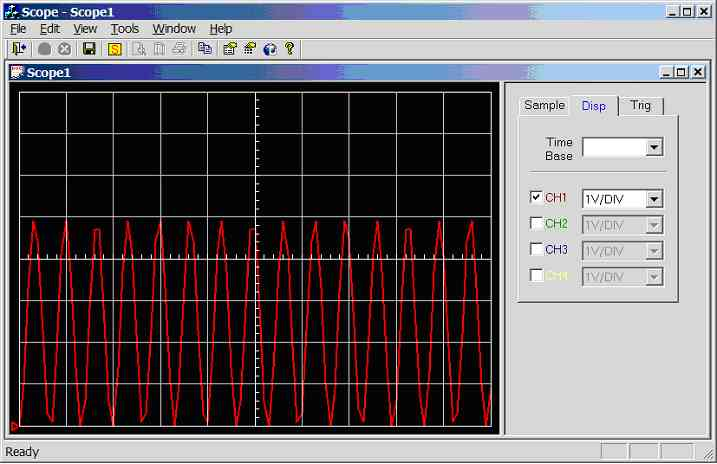Figure 11.3.2.3g. Screen dump of scope program (sample rate = 5000Hz, input wave = 750Hz sinewave 0-5V)

1000Hz sinewave (0-5V) connected to CH1 (AN0), see figure 11.3.2.3h. 0.5 divisions per cycle, hence the calculated frequency is 1/(0.5 *0.002) = 1000Hz, but distorted due to under sampling.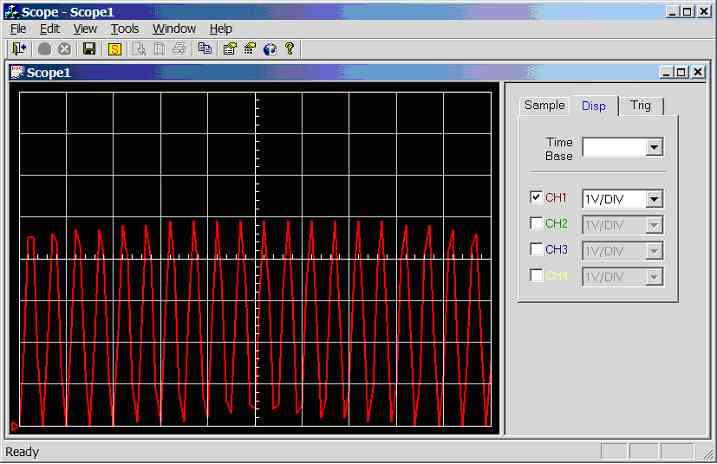Figure 11.3.2.3h. Screen dump of scope program (sample rate = 5000Hz, input wave = 1,000Hz sinewave 0-5V)

2500Hz sinewave (0-5V) connected to CH1 (AN0), see figure 11.3.2.3i. Under sampled.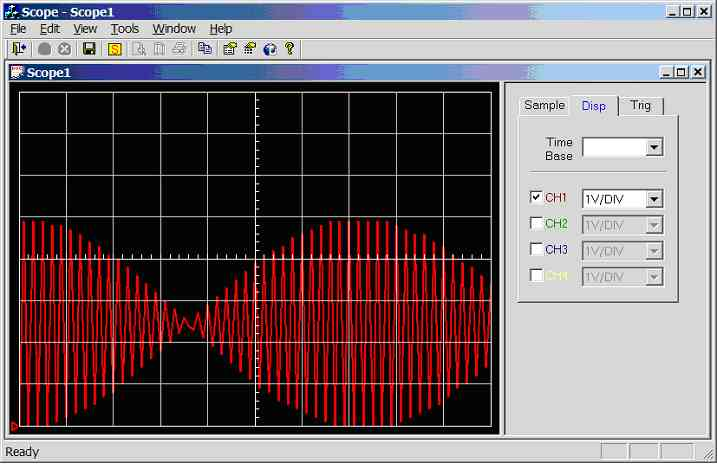Figure 11.3.2.3i. Screen dump of scope program (sample rate = 5000Hz, input wave = 2,500Hz sinewave 0-5V)

11.3.3. Test 10: Test External RAM chip

The circuit diagram shown in figure 11.3.3a was constructed on prototype board, with RS232 communication connected to laptop (COM1, 1000Mhz AMD processor, 256Mhz SDRAM, 8MB 3D accelerator card, running windows XP).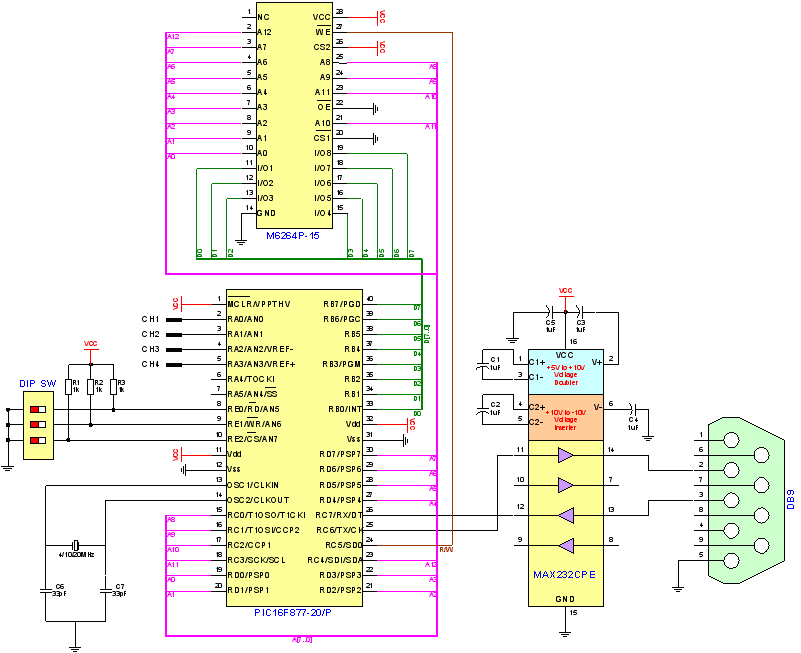Figure 11.3.3a. Test Circuit Diagram 3

Program mark10.c was compiled and loaded into the PIC. This program carries out three tests: -

1.      Fill all memory locations with 0xFFh and check.
2.      Fill all memory locations with 0x00h and check.
3.      Fill all memory locations with the LSB of the address and check.

RS232 communication is used to relay the results to the PC; the DOS based program test.exe used in RX terminal mode was used to receive and display the results.

Screen dump of the result is shown below: -
 RX Terminal ----------- Waiting for message (press a key to stop) TEST RAM CHIP...   Test 1 - Fill RAM with 0xFF ---> Passes = 7806, Fails = 386   Test 2 - Fill RAM with 0x00 ---> Passes = 8114, Fails = 78   Test 3 - Fill RAM with LSB of Address ---> Passes = 31, Fails = 8161   End of RAMTEST, Hopefully work can now start on storage mode

Clearly this test was a failure, it was discovered that there was a bug in the PIC program code (version 1.1). Recall that the DIP switches are connected to port E and in order to configure this port for TTL inputs, parallel slave mode is turn ON. It is clear from the PIC data sheet that this parallel slave mode also effects port D, which is connected to the address bus. Switching off parallel slave mode solved the problem, but created a new one, DIP switches cannot be read. The solution to this new problem was simple, before reading the DIP switches turn ON parallel slave mode, and after reading the DIP switches turn OFF parallel slave mode.

Screen dump of the result for the corrected mark10.c program is shown below: -
RX Terminal
-----------
Waiting for message (press a key to stop)
TEST RAM CHIP...

Test 1 - Fill RAM with 0xFF ---> Passes = 8192, Fails = 0

Test 2 - Fill RAM with 0x00 ---> Passes = 8192, Fails = 0

Test 3 - Fill RAM with LSB of Address ---> Passes = 8192, Fails = 0

End of RAMTEST, Hopefully work can now start on storage mode.

Clearly the RAM test was a success, and work can now start on the storage mode embedded program.

11.3.4. Test 11: 5V Regulator

The system powering circuit (figure 5.5a) was constructed on prototype board. The input voltage was varied from 8 to 20 VDC, while the output voltage was measured using a digital CRT storage oscilloscope. Output voltage was 5 VDC, throughout the test.

11.3.5. Test 12: Test 12: Analogue Circuitry

The analogue circuitry (figure 5.4a) circuit was constructed on proto-type board and tested. Signal generator was used to generate the input, and a digital CRT storage oscilloscope was used to check the output before connecting to the PIC ADC. The test was not perfect, as the scale was incorrect. For example a -2.5 to 2.5 volt sine-wave was connected to the -2.5 to 2.5 input, the output was 1 to 5 volts and not 0 to 5 volts as expected. Allow this could be software corrected; it makes since to play around with the resistor values to try and improve this. (Perhaps variable resistors may have to be used for calibration purposes).

Note a switch mode DC to DC converter was used to converter 5 Vdc to -15 and 15V. Hence the entire circuit was power from one supply. Note the diodes successfully stopped the output voltage getting too low or too high.

11.3.6. Conclusion

Evidently the scope program can view up to four channels at a sample rate of 1kHz and one channel at a sample rat of 5kHz in real-time. Clearly there is a triggering problem as it was difficult to obtain a stable trace when triggering off another channel, for example when triggering off channel 1, all other channels were constantly scrolling across the screen. When the same signal is inputted into all four channels, it is now possible to get a stable trigger (e.g. waveforms related), but waveforms of different frequencies was a problem.

Clearly storage mode operation is now possible, as the RAM chip was successfully tested. The first step might be to use the real-time frame structure, storing up to 4000 reading into RAM and then cycling through them transmitting them to the PC in one large block. This is a quick and easy test, next step is actually writing the storage mode software and then the control protocol.